# Change Nickname

Current Nickname:

v4.0
Search
中文EN
v4.0

# LINE

## Overview

LINE is short for Large-scale Information Network Embedding. LINE is a graph embedding model, it uses the BFS neighborhood information (first-order or second-order proximity) of nodes to construct training samples, and train node embeddings by the method similar to Node2Vec. LINE is able to scale to very large, arbitrary types of networks, it was proposed by J. Tang et al. of Microsoft in 2015.

Related material of the algorithm:

## Basic Concept

### First-order Proximity and Second-order Proximity

First-order proximity between nodes in the graph depends on the connectivity, that is, the larger the edge weight (edge weight equals to 1 for unweighted graph; negative weight is not considered here) between nodes, the higher the first-order proximity they have. In case there is no link between two nodes, their first-order proximity is 0. First-order proximity reflects the local structure.

Second-order proximity reflects the global structure, and it is determined by the common neighbors two nodes share. The general notion of the second-order proximity is actually easy to understand, for instance, in social network, the more friends two people have in common, the higher the connection between the two people. In case two nodes have no common neighbors, their second-order proximity is 0.

As shown in the graph below, the thickness of edge represents the weight size. As the weight of the edge between nodes 6 and 7 is large, i.e., they have a high first-order proximity, they should be represented closely to each other in the embedded space. On the other hand, though there is no edge between nodes 5 and 6, they share many common neighbors, i.e., they have a high second-order proximity and therefore should also be represented closely to each other.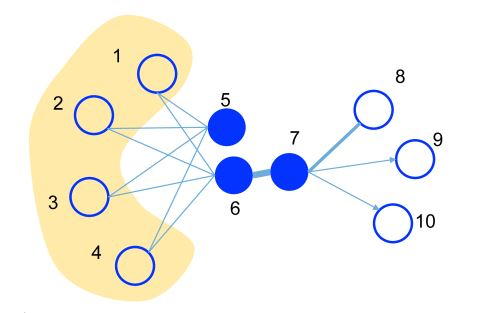### Model Description

We describe the LINE model to preserve the first-order proximity and second-order proximity separately, and then introduce a simple way to combine the two proximity.

#### LINE with First-order Proximity

For graph G = (V, E), to model the first-order proximity, LINE defines the joint probability between nodes vi and vj as follows: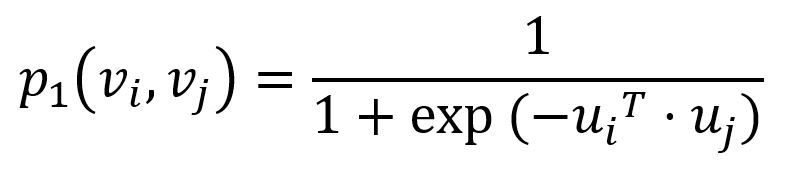where u is the low-dimensional vector representation of node. Empirical joint probability between node vi and vj can be defined as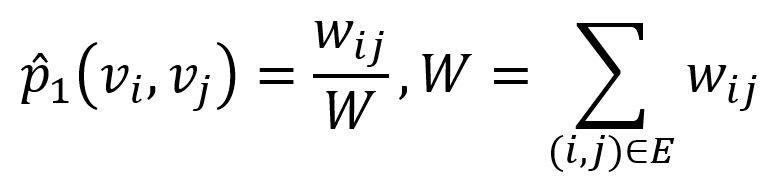where wij denotes the edge weight between nodes vi and vj.

To preserve the first-order proximity, a straightforward way is to minimize the difference between the two distributions (i.e., the value predicted by the model is close to the actual value). LINE adopts the KL (Kullback-Leibler) divergence to measure the difference (constants are omitted):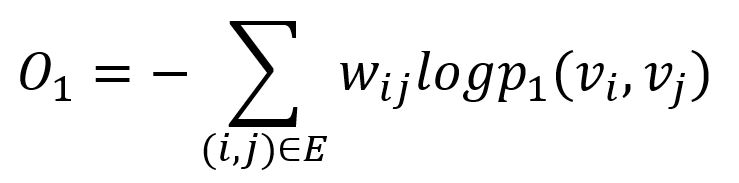Note that first-order proximity is only applicable for undirected graphs, not for directed graphs.

#### LINE with Second-order Proximity

In graph, each node plays two roles: the node itself and a specific 'context' of other node. Nodes with similar distributions over the 'contexts' are assumed to have second-order proximity. We define the probability of context vj generated by node vi as follows (without loss of generality, we assume it is directed):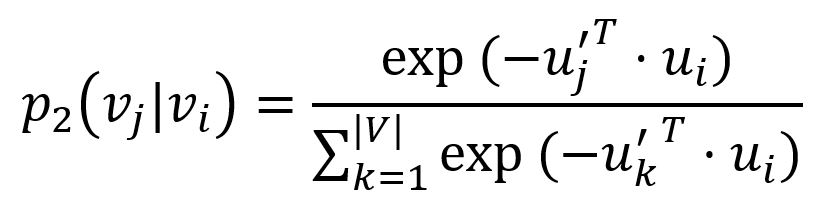where u' is the representation of node when it is treated as a 'context', |V| is the number of nodes or 'contexts' in the graph. The corresponding empirical probability can be defined as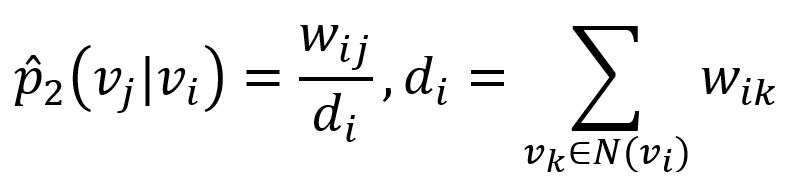where wij denotes the edge weight between nodes vi and vj, di is the out-degree of node vi.

Similarly, LINE adopts the KL (Kullback-Leibler) divergence to measure the difference (constants are omitted) between these two probability distributions: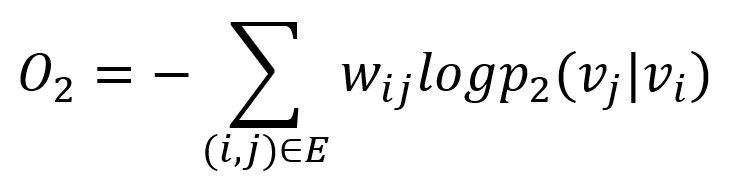Second-order proximity is applicable for both directed and undirected graphs.

#### Combining First-order and Second-order Proximities

To embed the networks by preserving both the first-order and second-order proximity, a simple and effective way we find in practice is to train the LINE model which preserves the first-order proximity and second-order proximity separately and then concatenate the embeddings trained by the two methods for each vertex.

### Model Optimization

Optimizing objective O2 is computationally expensive, which requires the summation over the entire set of nodes when calculating the conditional probability p2(·|vi). To address this problem, LINE adopts the approach of negative sampling. More specifically, it specifies the following objective function for each edge from node vi to vj: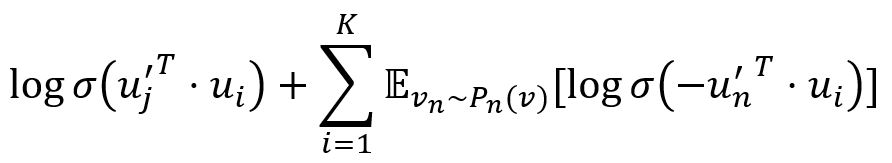where `σ` is the sigmoid function. The first term models the observed edges, the second term models the negative edges drawn from the noise distribution and K is the number of negative edges. We set Pn(v) ∝ dv3/4, and dv is the out degree of node v.

## Special Case

### Lonely Node, Disconnected Graph

Lonely node has no adjacent node, hence it has no first-order or second-order proximity with any other nodes.

Nodes only has first-order or second-order proximity with nodes in the same connected component.

### Self-loop Edge

Self-loop edges of node have nothing to do with the first-order or second-order proximity the node has with other nodes.

### Directed Edge

First-order proximity is only applicable for undirected graphs, not for directed graphs. Second-order proximity is applicable for both directed and undirected graphs.

## Command and Configuration

• Command：`algo(line)`
• Configurations for the parameter `params()`:
Name Type
Default
Specification
Description
edge_schema_property []`@<schema>?.<property>` / Numeric edge property, LTE needed Edge weight property/properties, schema can be either carried or not; edge without any specified property does not participate in the calculation; degree is unweighted if not set
dimension int / >=1 Dimension of node embedding vector
resolution int / >=1 Such as 10, 100
learning_rate float / (0,1) Initial learning rate, it decreases until reaches `min_learning_rate` with increase in training iterations
min_learning_rate float / (0,`learning_rate`) Minimum learning rate
neg_num int / >=0 Number of negative samples, it is suggested 0~10
train_total_num int / >=1 Total training times
train_order int 1 1 or 2 Type of proximity, 1 means first-order proximity, 2 means second-order proximity
limit int -1 >=-1 Number of results to return; return all results if sets to -1 or not set

## Algorithm Execution

#### 1. File Writeback

Configuration Data in Each Row
filename `_id`,`embedding_result`

#### 2. Property Writeback

Not supported by this algorithm.

#### 3. Statistics Writeback

This algorithm has no statistics.

### Direct Return

Alias Ordinal Type
Description
Column Name
0 []perNode Array of UUIDs and embeddings of nodes `_uuid`, `embedding_result`

### Streaming Return

Alias Ordinal Type
Description
Column Name
0 []perNode Array of UUIDs and embeddings of nodes `_uuid`, `embedding_result`

### Real-time Statistics

This algorithm has no statistics.